# Some 2-d plot questions

1. How draw 2 or more functions on one image to compare?
2. On AxisY (if y is small) I see 1,2,3 but I don't know if 1 means 1e-6, 1e-8 or 1e-10. Is possible draw this information on image?
3. For very small y , is order of magnitude 1e-16, are visible rounding errors. Is possible draw with more precision than double? I see "fast_callable" example but this not works in my case.

Example for question 3.

Goal: plot p(x)-exp(x) where p is a Chebyshev approximation of exp of degree > 10.

For a concrete example, let us use the degree 11 Chebyshev approximation for the exponential.

We rescale the x-axis from [-1,1] to [0,1], and thus change exp(x) to exp(x/2 + 1/2).

We use this Chebyshev approximation.

f(x) = (1.648721270700127734695199 + 0.8243606353500639704213267*x + 0.2060901588375456897278408*x^2 + 0.03434835980625555348009372*x^3 + 0.004293544975435477755738488*x^4 + 0.0004293544975550920330337524*x^5 + 3.577954294118110098524956e-05*x^6 + 2.555681612268918733452545e-06*x^7 + 1.597272498430465439987321e-07*x^8 + 8.873762489265668731711247e-09*x^9 + 4.462222789173961848447732e-10*x^10 + 2.027323563290198480646098e-11*x^11)


Here is the plot we get:

plot(f(x) - exp(x/2 + 1/2), x)


or, after applying some of the hints regarding question 2:

plot(f(x) - exp(x/2 + 1/2), x, frame=True, figsize=(7, 2))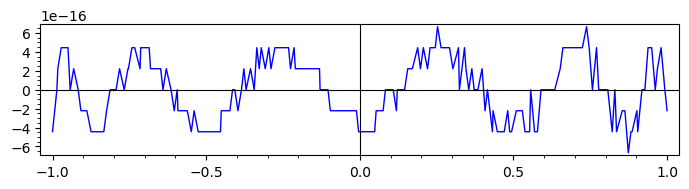edit retag close merge delete

For 1, use P+Q where P and Q are two plots. Or use a sequence of functions as argument of plot.

For question 3, a concrete example would make it easier to explore the question.

To give a general suggestion, computing in arbitrary precision with Arb, via RealBallField or ComplexBallField, might be a way to alleviate such problems.

3.Example: plot(p(x)-exp(x),x) where p(x) = Chebyshev approximation of exp(x) for degree>10

Please provide complete code, including defining p(x), for anyone to copy-paste.

This saves effort and time when exploring the question.

Sort by » oldest newest most voted

## Plot several functions together

This answers question 1, following @FrédéricC's suggestions.

Summing individual plots:

sage: pcos = plot(cos, (-4, 6), color='steelblue')
sage: psin = plot(sin, (-4, 6), color='purple')
sage: (pcos + psin).show(aspect_ratio=1)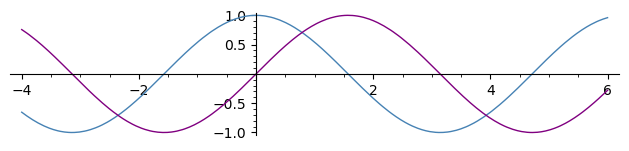Plotting several functions together (see SageMath documentation on 2D plotting):

sage: plot([x*exp(-n*x^2)/.4 for n in [1 .. 5]], (0, 2), aspect_ratio=.5)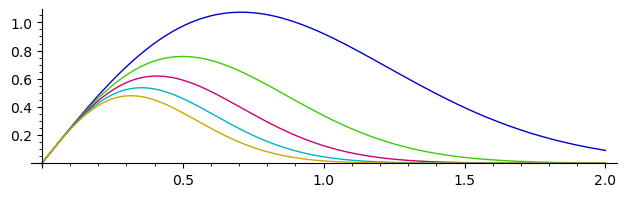## Tick label rendering bug

This bug was also reported as

Solving that problem is now tracked at

Until the bug is fixed, a workaround is to plot with a frame, which will indicate the scaling.

Compare:

sage: p = plot(lambda x: 8e6*x); p
sage: q = plot(lambda x: 8e6*x, frame=True); q
sage: graphics_array([p, q]).show(figsize=(8, 4))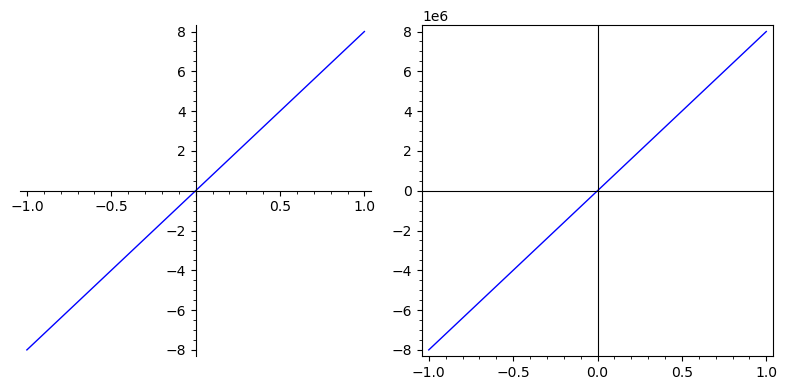Or you could use the get_minmax_data method to get the xmin, xmax, ymin, ymax, and somehow add them to the picture as text.

sage: d = p.get_minmax_data()
sage: xa, xb, ya, yb = d['xmin'], d['xmax'], d['ymin'], d['ymax']
sage: xc, yc = mean([xa, xa, xb]), mean([ya, ya, yb])
sage: txa = text(f"xmin:\n{xa}", (xa, yc), horizontal_alignment='left')
sage: txb = text(f"xmax:\n{xb}", (xb, yc), horizontal_alignment='right')
sage: tya = text(f"ymin:\n{ya}", (xc, ya), vertical_alignment='bottom')
sage: tyb = text(f"ymax:\n{yb}", (xc, yb), vertical_alignment='top')
sage: pt = p + sum([txa, txb, tya, tyb])
sage: pt
Launched png viewer for Graphics object consisting of 5 graphics primitives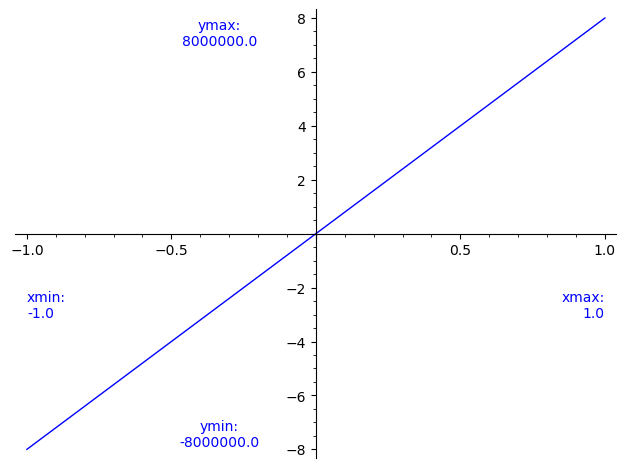## Rounding errors in floating-point calculations

### Problematic plot

The exponential function on [0, 1], rescaled to [-1, 1].

sage: h(x) = exp(x/2 + 1/2)


Its Chebyshev approximation of order 11:

sage: f(x) = 1.648721270700127734695199 + 0.8243606353500639704213267*x + 0.2060901588375456897278408*x^2 + 0.03434835980625555348009372*x^3 + 0.004293544975435477755738488*x^4 + 0.0004293544975550920330337524*x^5 + 3.577954294118110098524956e-05*x^6 + 2.555681612268918733452545e-06*x^7 + 1.597272498430465439987321e-07*x^8 + 8.873762489265668731711247e-09*x^9 + 4.462222789173961848447732e-10*x^10 + 2.027323563290198480646098e-11*x^11


The quality-of-approximation function: their difference:

sage: g(x) = f(x) - h(x)


The problematic plot:

sage: pg = plot(g, color='steelblue')
sage: pg.show(frame=True, figsize=(7, 2))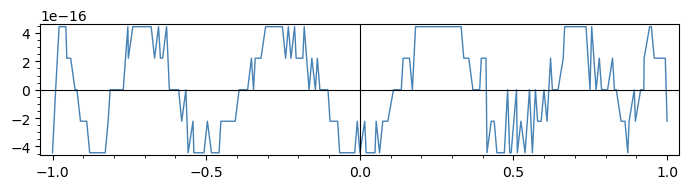### Plotting uses floating-point computations!

The plot in the question seems to be taking only 7 y-values.

This plot's goal is to visualise a quality of approximation by plotting the difference f(x) - h(x) of two almost-equal functions (f is an approximation of h of high quality).

Of course, this means the function g = f - h takes extremely small values. The way they are computed becomes important!

Knowing how the plot command works helps understand what is going on: it samples 200 floating-point values in the plotting interval and computes the value of the function at these points. These are floating-point computations, with 53 bits of precision, which is roughly 15 or 16 decimal digits.

Here, both f and h have values that are roughly on the order of one, and their difference is on the order of 1e-16. (Furthermore f itself is a polynomial, so each f(x) is a linear combination of twelve powers of x.)

In the end each g(x) is the sum of terms of various sizes, summed in a certain order, resulting in a value very close to the precision of some of the numbers being summed.

It becomes hard to put much trust our output.

### Arb to the rescue

A general suggestion for dealing with precision issues is to use Arb for arbitrary precision calculations.

In Sage, Arb can be used via RealBallField and ComplexBallField.

The Sage documentation and Ask Sage have a few examples.

For the purpose of this question, we can use evaluation of polynomials with integer or rational coefficients on real or complex balls. This is a recent feature of Arb, even more recently available in Sage (thanks to the work at Sage Trac ticket 30652) In fact it is only part of Sage since version 9.3.beta0.

Here is one way to use that.

Define the coefficients of the Chebyshev polynomial approximation.

sage: cc = (1.648721270700127734695199,
....:       0.8243606353500639704213267,
....:       0.2060901588375456897278408,
....:       0.03434835980625555348009372,
....:       0.004293544975435477755738488,
....:       0.0004293544975550920330337524,
....:       3.577954294118110098524956e-05,
....:       2.555681612268918733452545e-06,
....:       1.597272498430465439987321e-07,
....:       8.873762489265668731711247e-09,
....:       4.462222789173961848447732e-10,
....:       2.027323563290198480646098e-11)


Turn them into rationals:

sage: ccc = [QQ(c.as_integer_ratio()) for c in cc]


Define the polynomial ring over QQ with variable x:

sage: P = QQ['x']


Define the Chebyshev approximation as a polynomial over QQ:

sage: ff = P(ccc)


Define the quality-of-approximation function:

sage: def gg(x):
....:     return ff(x) - exp(x/2 + 1/2)


Define a real ball field with sufficient precision (here we take 1000 bits).

sage: R = RealBallField(1000)


Define an alternative version of the quality-of-approximation function using real balls:

sage: def ggg(x):
....:     return gg(R(x))


Plot:

sage: pggg = plot(ggg, color='purple)
sage: pggg.show(frame=True, figsize=(7, 2))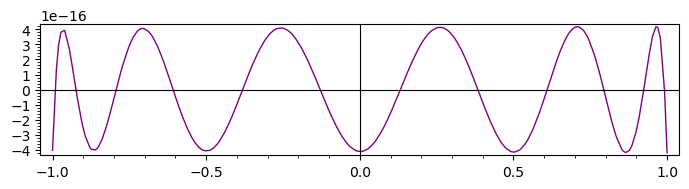Compare to the original plot:

sage: p = plot(f(x) - exp(x/2 + 1/2), x, frame=True, figsize=(7, 2))
sage: q = plot(gg, color='steelblue', frame=True, figsize=(7, 2))
sage: (p + q).show(figsize=(7, 2))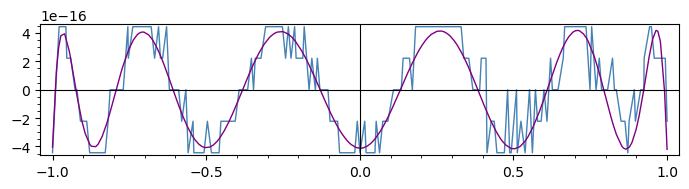This comparison shows that despite its jaggedness, the low-resolution plot was still giving an indication of the variations of the difference between the approximating and the approximated functions.

more

what is ff? if I replace ff by f, I get empty plot. verbose 0 (3835: plot.py, generate_plot_points) Last error message: 'unsupported operand parent(s) for *: 'Real ball field with 1000 bits of precision' and 'Real Field with 84 bits of precision''